Скачать презентацию Optimisation The general problem Want to minimise

9489677161afdb0bdac22d5ef18e532c.ppt

• Количество слайдов: 23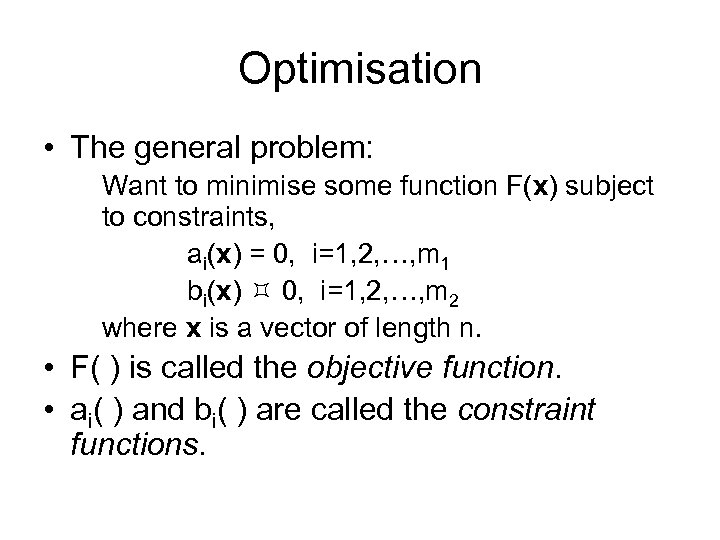Optimisation • The general problem: Want to minimise some function F(x) subject to constraints, ai(x) = 0, i=1, 2, …, m 1 bi(x) 0, i=1, 2, …, m 2 where x is a vector of length n. • F( ) is called the objective function. • ai( ) and bi( ) are called the constraint functions.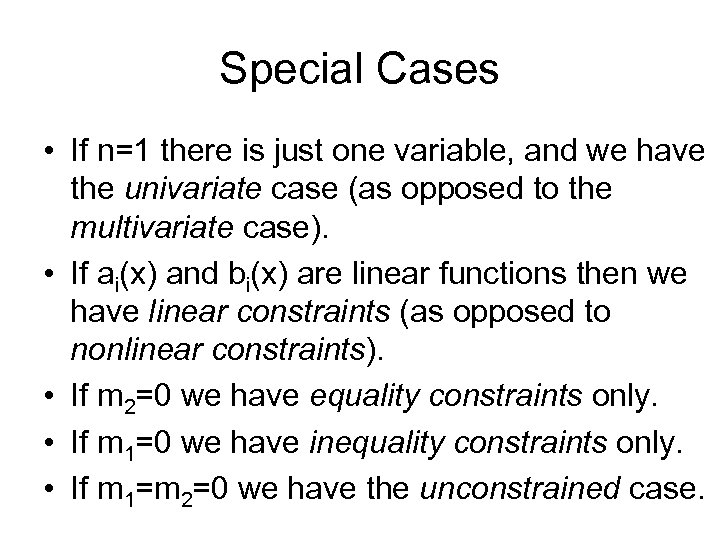Special Cases • If n=1 there is just one variable, and we have the univariate case (as opposed to the multivariate case). • If ai(x) and bi(x) are linear functions then we have linear constraints (as opposed to nonlinear constraints). • If m 2=0 we have equality constraints only. • If m 1=0 we have inequality constraints only. • If m 1=m 2=0 we have the unconstrained case.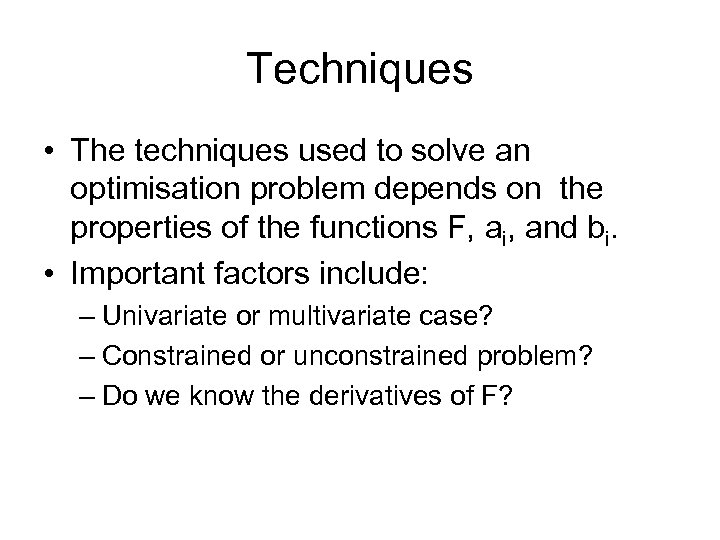Techniques • The techniques used to solve an optimisation problem depends on the properties of the functions F, ai, and bi. • Important factors include: – Univariate or multivariate case? – Constrained or unconstrained problem? – Do we know the derivatives of F?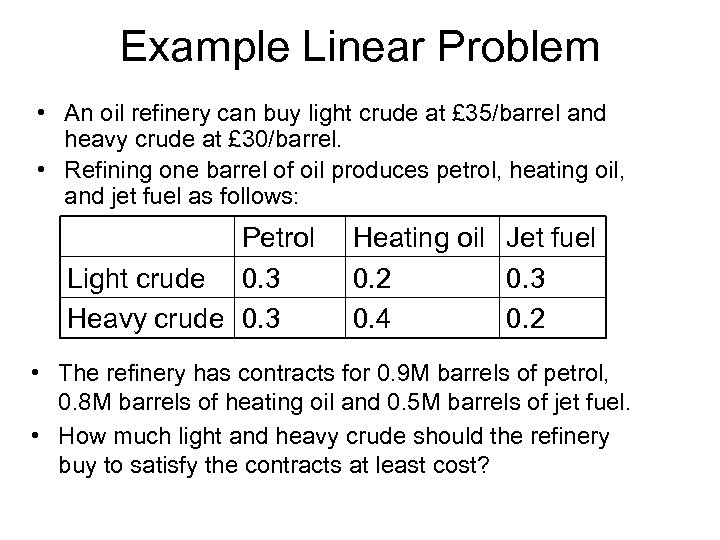Example Linear Problem • An oil refinery can buy light crude at £ 35/barrel and heavy crude at £ 30/barrel. • Refining one barrel of oil produces petrol, heating oil, and jet fuel as follows: Petrol Light crude 0. 3 Heavy crude 0. 3 Heating oil Jet fuel 0. 2 0. 3 0. 4 0. 2 • The refinery has contracts for 0. 9 M barrels of petrol, 0. 8 M barrels of heating oil and 0. 5 M barrels of jet fuel. • How much light and heavy crude should the refinery buy to satisfy the contracts at least cost?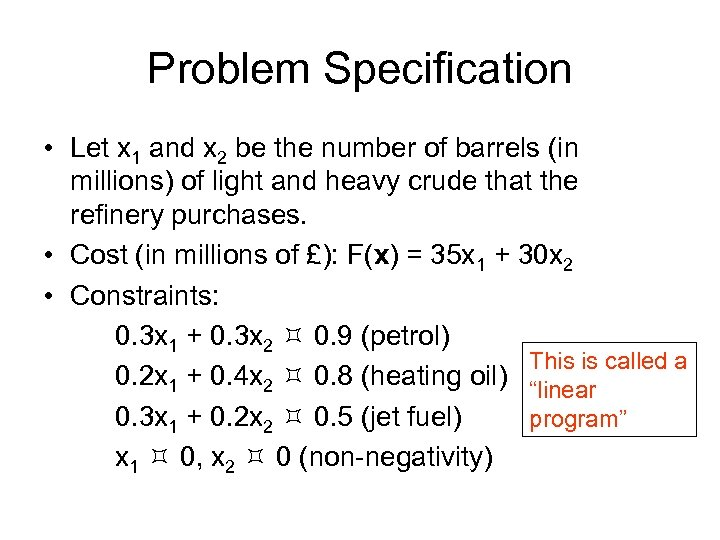Problem Specification • Let x 1 and x 2 be the number of barrels (in millions) of light and heavy crude that the refinery purchases. • Cost (in millions of £): F(x) = 35 x 1 + 30 x 2 • Constraints: 0. 3 x 1 + 0. 3 x 2 0. 9 (petrol) This is called a 0. 2 x 1 + 0. 4 x 2 0. 8 (heating oil) “linear 0. 3 x 1 + 0. 2 x 2 0. 5 (jet fuel) program” x 1 0, x 2 0 (non-negativity)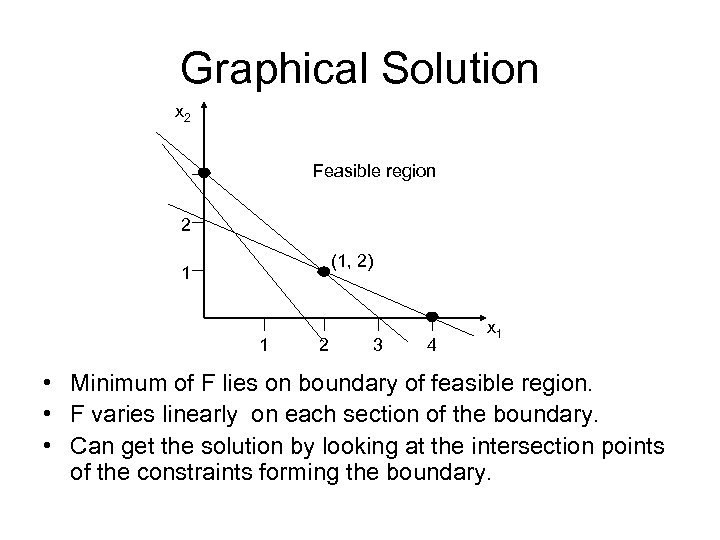Graphical Solution x 2 Feasible region 2 (1, 2) 1 1 2 3 4 x 1 • Minimum of F lies on boundary of feasible region. • F varies linearly on each section of the boundary. • Can get the solution by looking at the intersection points of the constraints forming the boundary.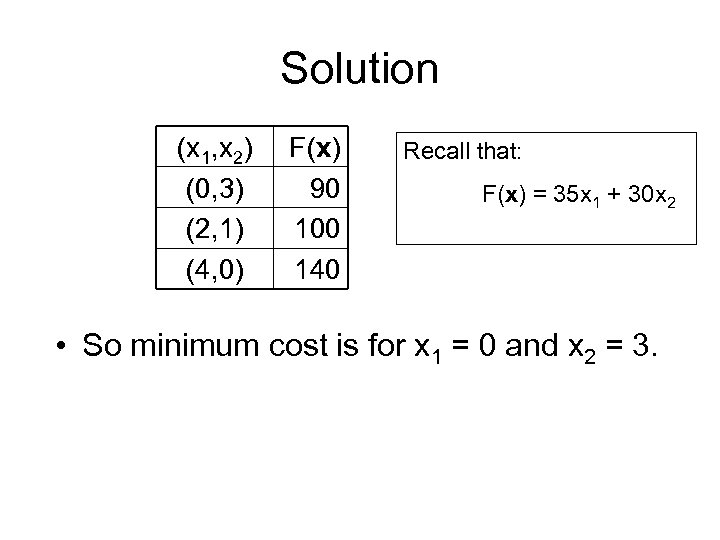Solution (x 1, x 2) (0, 3) (2, 1) (4, 0) F(x) 90 100 140 Recall that: F(x) = 35 x 1 + 30 x 2 • So minimum cost is for x 1 = 0 and x 2 = 3.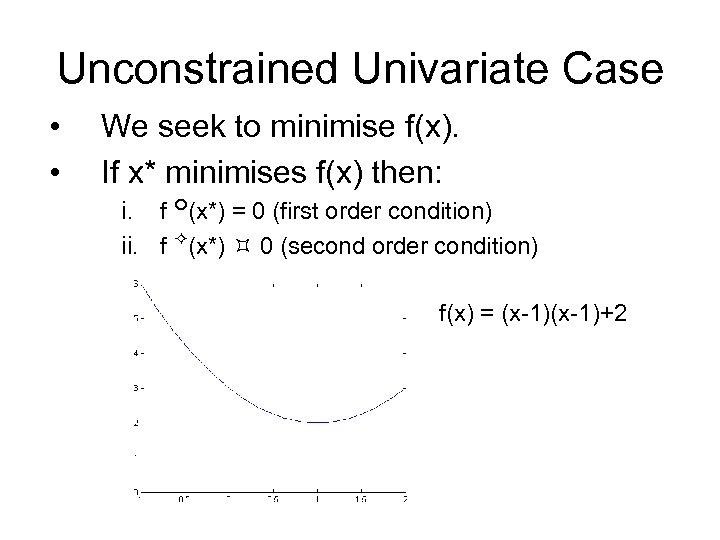Unconstrained Univariate Case • • We seek to minimise f(x). If x* minimises f(x) then: i. f (x*) = 0 (first order condition) ii. f (x*) 0 (second order condition) f(x) = (x-1)+2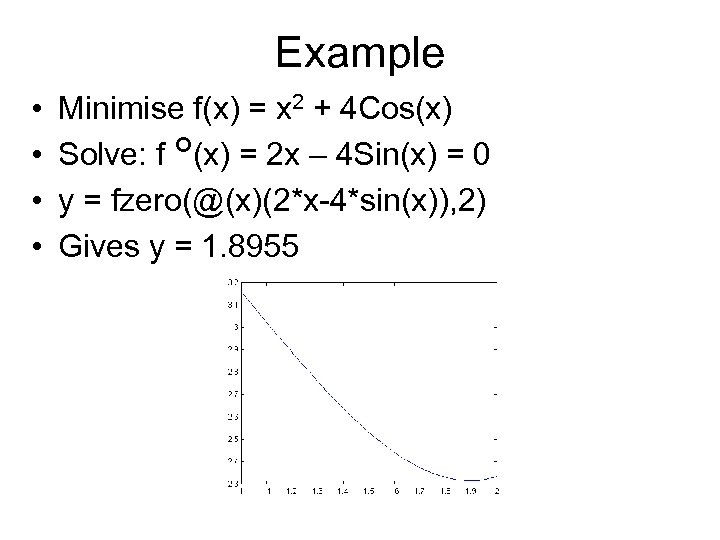Example • • Minimise f(x) = x 2 + 4 Cos(x) Solve: f (x) = 2 x – 4 Sin(x) = 0 y = fzero(@(x)(2*x-4*sin(x)), 2) Gives y = 1. 8955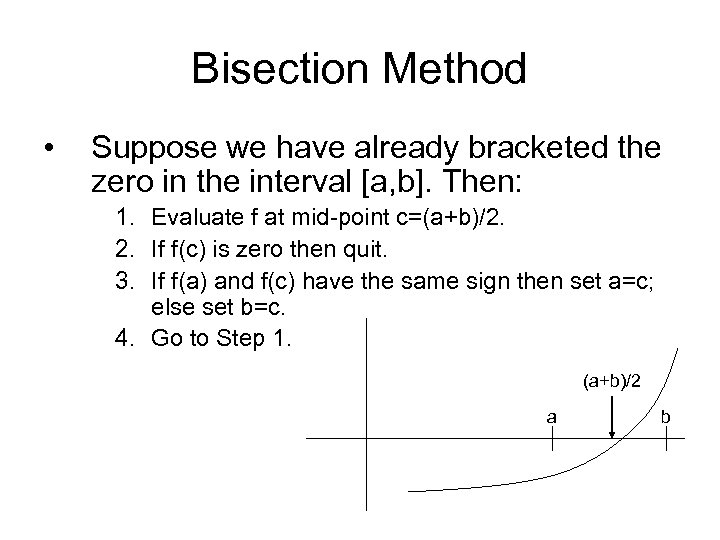Bisection Method • Suppose we have already bracketed the zero in the interval [a, b]. Then: 1. Evaluate f at mid-point c=(a+b)/2. 2. If f(c) is zero then quit. 3. If f(a) and f(c) have the same sign then set a=c; else set b=c. 4. Go to Step 1. (a+b)/2 a b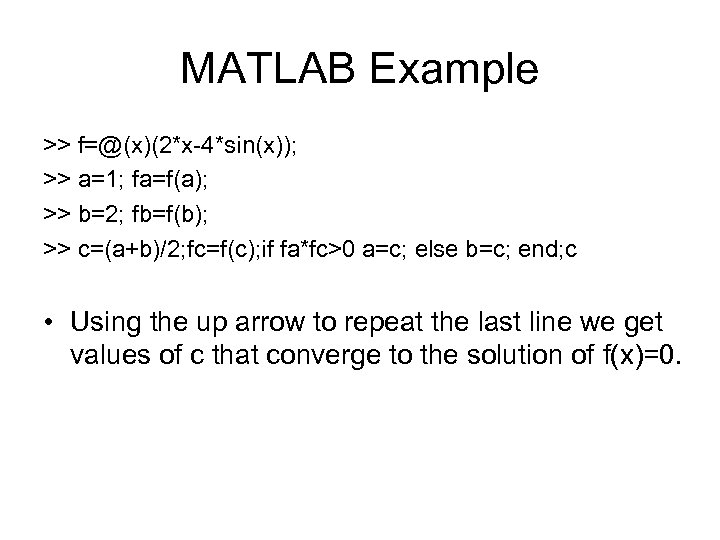MATLAB Example >> f=@(x)(2*x-4*sin(x)); >> a=1; fa=f(a); >> b=2; fb=f(b); >> c=(a+b)/2; fc=f(c); if fa*fc>0 a=c; else b=c; end; c • Using the up arrow to repeat the last line we get values of c that converge to the solution of f(x)=0.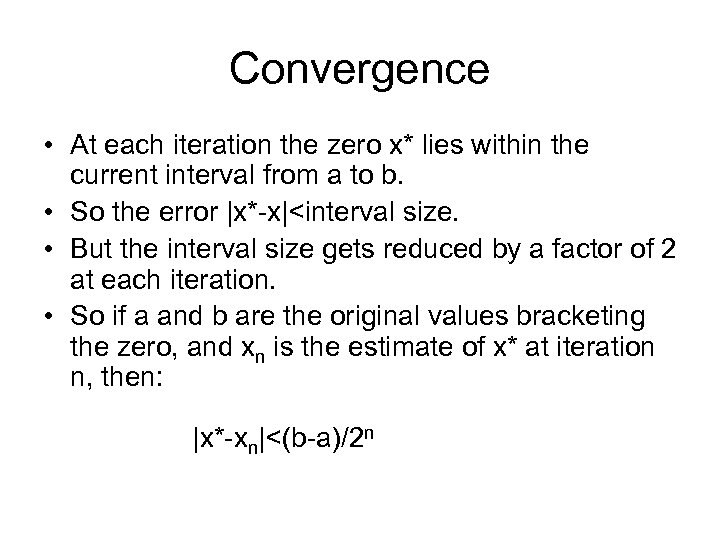Convergence • At each iteration the zero x* lies within the current interval from a to b. • So the error |x*-x|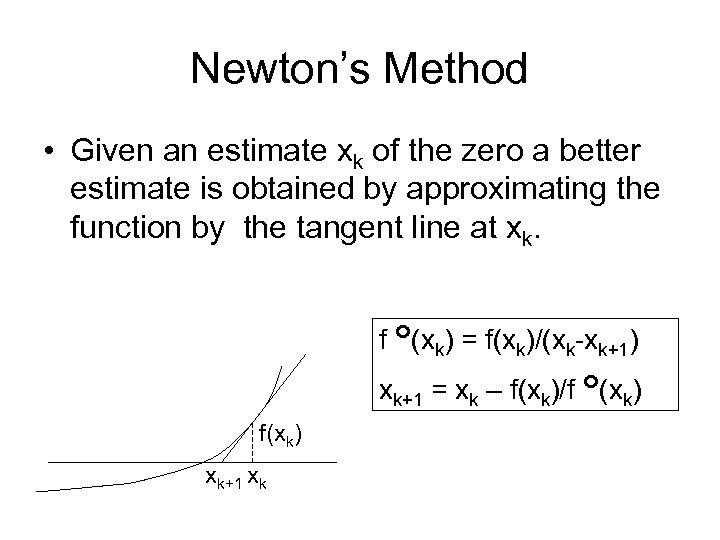Newton’s Method • Given an estimate xk of the zero a better estimate is obtained by approximating the function by the tangent line at xk. f (xk) = f(xk)/(xk-xk+1) xk+1 = xk – f(xk)/f (xk) f(xk) xk+1 xk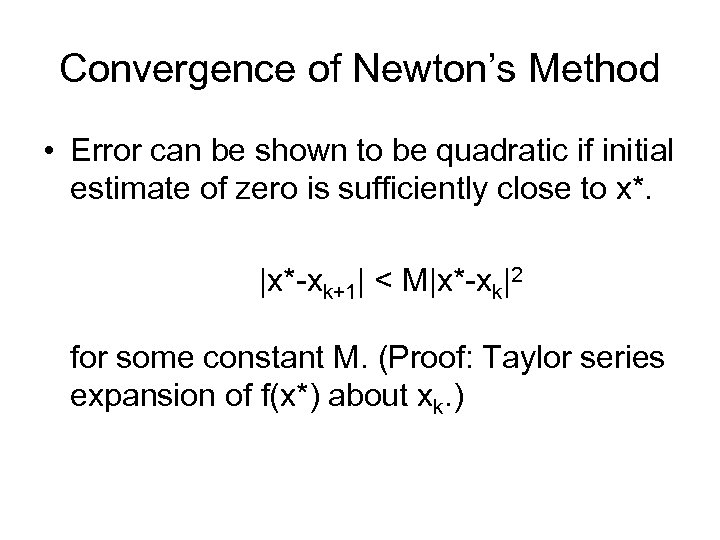Convergence of Newton’s Method • Error can be shown to be quadratic if initial estimate of zero is sufficiently close to x*. |x*-xk+1| < M|x*-xk|2 for some constant M. (Proof: Taylor series expansion of f(x*) about xk. )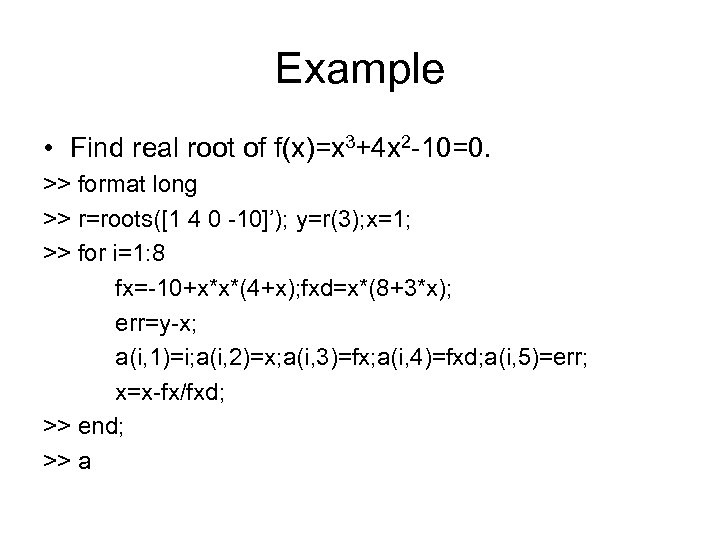Example • Find real root of f(x)=x 3+4 x 2 -10=0. >> format long >> r=roots([1 4 0 -10]’); y=r(3); x=1; >> for i=1: 8 fx=-10+x*x*(4+x); fxd=x*(8+3*x); err=y-x; a(i, 1)=i; a(i, 2)=x; a(i, 3)=fx; a(i, 4)=fxd; a(i, 5)=err; x=x-fx/fxd; >> end; >> a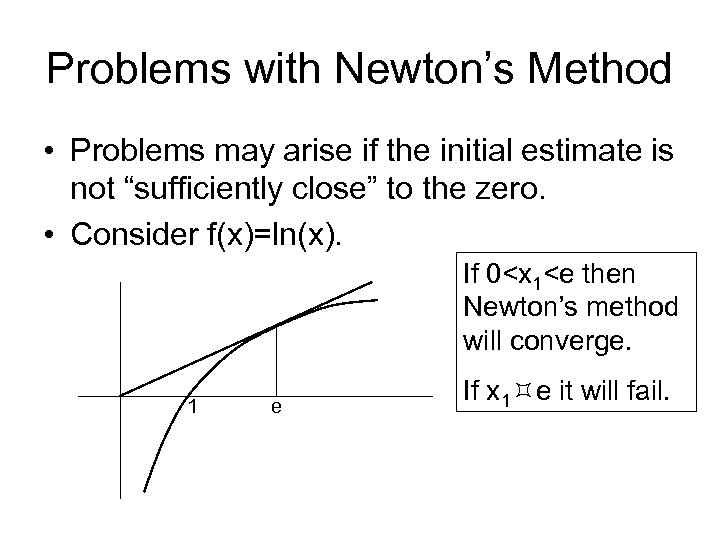Problems with Newton’s Method • Problems may arise if the initial estimate is not “sufficiently close” to the zero. • Consider f(x)=ln(x). If 0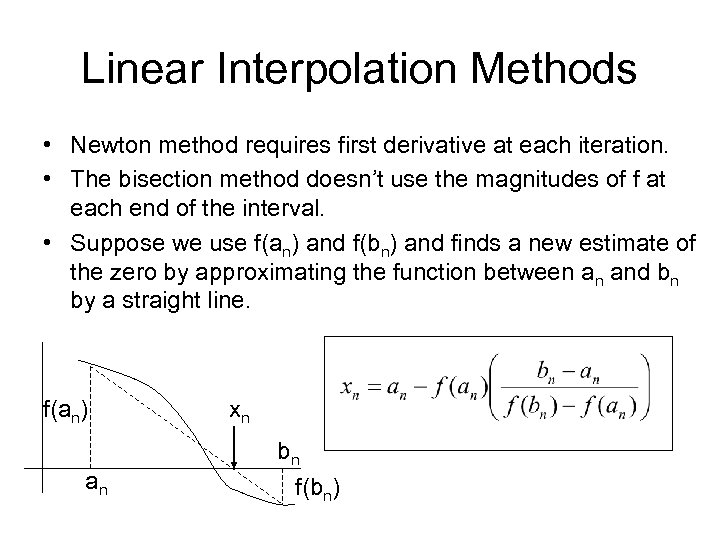Linear Interpolation Methods • Newton method requires first derivative at each iteration. • The bisection method doesn’t use the magnitudes of f at each end of the interval. • Suppose we use f(an) and f(bn) and finds a new estimate of the zero by approximating the function between an and bn by a straight line. f(an) an xn bn f(bn)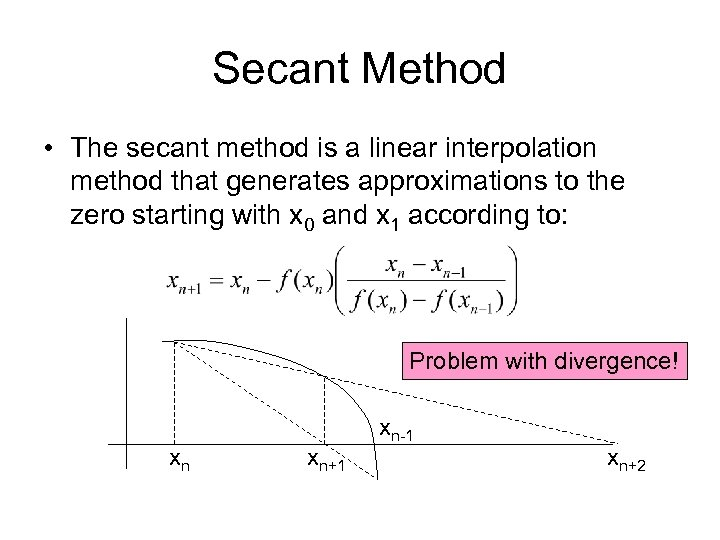Secant Method • The secant method is a linear interpolation method that generates approximations to the zero starting with x 0 and x 1 according to: Problem with divergence! xn xn+1 xn-1 xn+2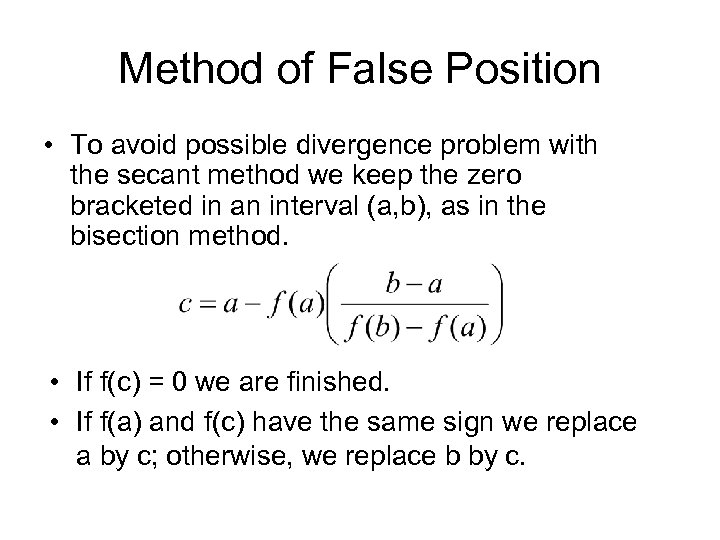Method of False Position • To avoid possible divergence problem with the secant method we keep the zero bracketed in an interval (a, b), as in the bisection method. • If f(c) = 0 we are finished. • If f(a) and f(c) have the same sign we replace a by c; otherwise, we replace b by c.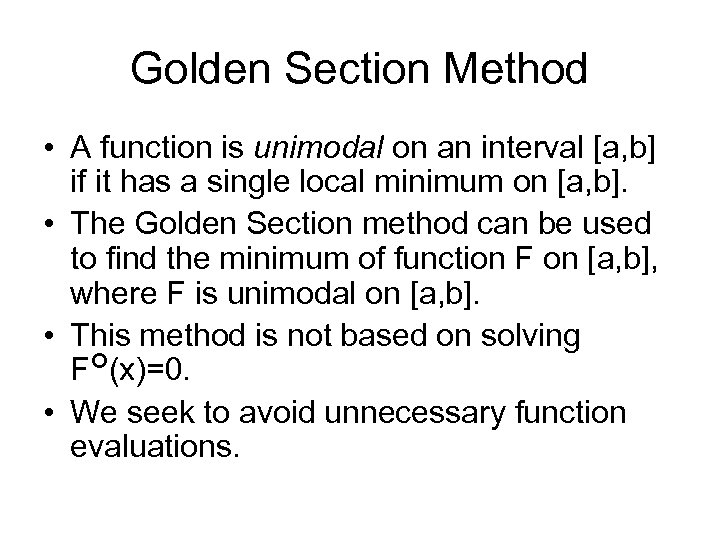Golden Section Method • A function is unimodal on an interval [a, b] if it has a single local minimum on [a, b]. • The Golden Section method can be used to find the minimum of function F on [a, b], where F is unimodal on [a, b]. • This method is not based on solving F (x)=0. • We seek to avoid unnecessary function evaluations.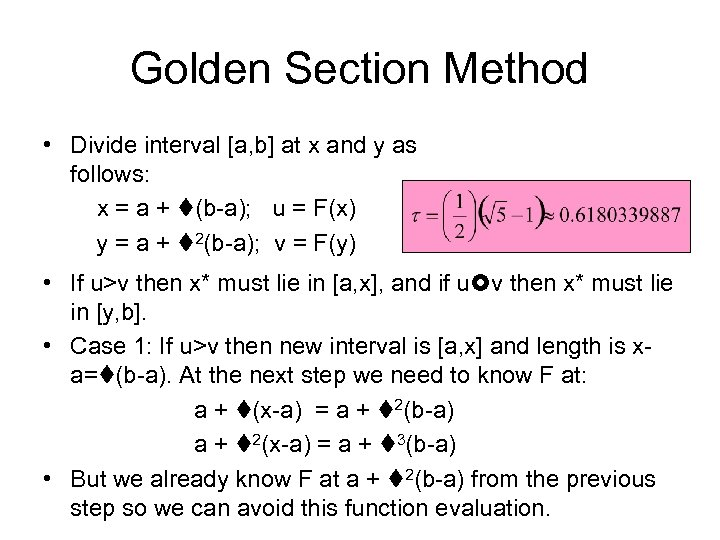Golden Section Method • Divide interval [a, b] at x and y as follows: x = a + (b-a); u = F(x) y = a + 2(b-a); v = F(y) • If u>v then x* must lie in [a, x], and if u v then x* must lie in [y, b]. • Case 1: If u>v then new interval is [a, x] and length is xa= (b-a). At the next step we need to know F at: a + (x-a) = a + 2(b-a) a + 2(x-a) = a + 3(b-a) • But we already know F at a + 2(b-a) from the previous step so we can avoid this function evaluation.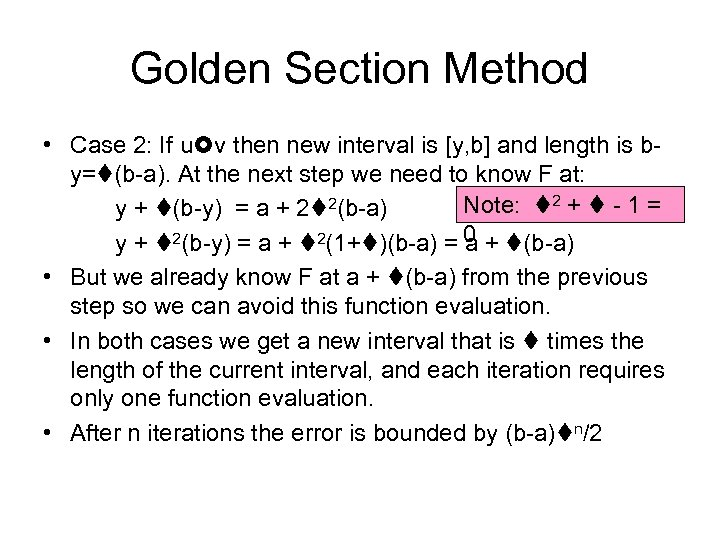Golden Section Method • Case 2: If u v then new interval is [y, b] and length is by= (b-a). At the next step we need to know F at: Note: 2 + - 1 = y + (b-y) = a + 2 2(b-a) y + 2(b-y) = a + 2(1+ )(b-a) = 0 + (b-a) a • But we already know F at a + (b-a) from the previous step so we can avoid this function evaluation. • In both cases we get a new interval that is times the length of the current interval, and each iteration requires only one function evaluation. • After n iterations the error is bounded by (b-a) n/2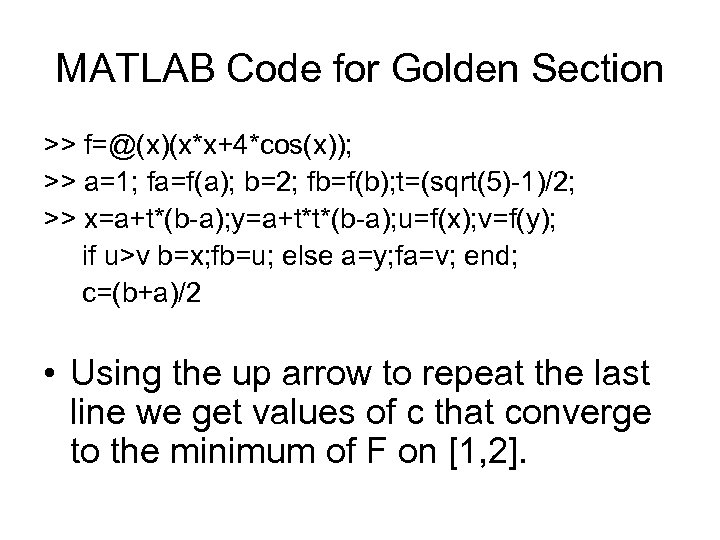MATLAB Code for Golden Section >> f=@(x)(x*x+4*cos(x)); >> a=1; fa=f(a); b=2; fb=f(b); t=(sqrt(5)-1)/2; >> x=a+t*(b-a); y=a+t*t*(b-a); u=f(x); v=f(y); if u>v b=x; fb=u; else a=y; fa=v; end; c=(b+a)/2 • Using the up arrow to repeat the last line we get values of c that converge to the minimum of F on [1, 2].